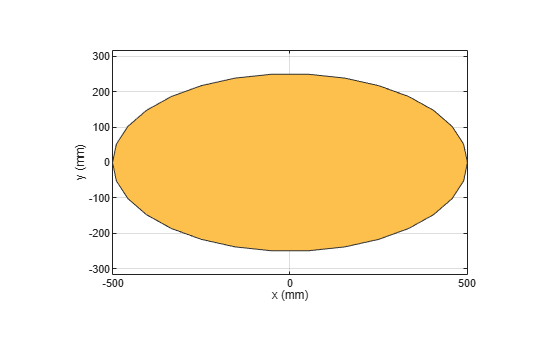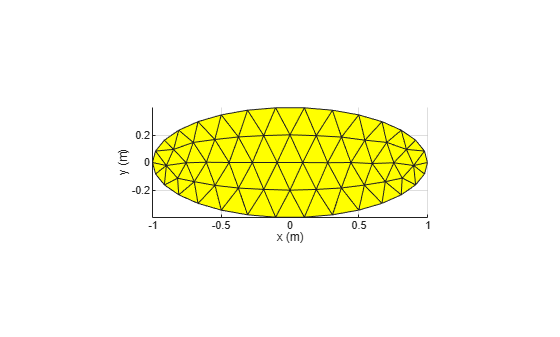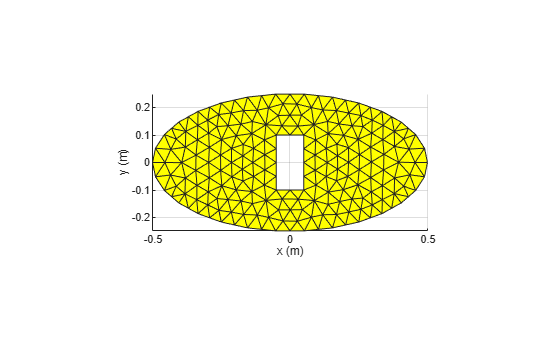# antenna.Ellipse

Create ellipse centered at origin on X-Y plane

## Description

Use the `antenna.Ellipse` object to create an ellipse centered at the origin on the X-Y plane.

## Creation

### Syntax

``ellipse = antenna.Ellipse``
``ellipse = antenna.Ellipse(Name,Value)``

### Description

example

````ellipse = antenna.Ellipse` creates an ellipse centered at the origin on the X-Y plane.```

example

````ellipse = antenna.Ellipse(Name,Value)` sets properties using one or more name-value pair arguments. For example, ```ellipse = antenna.Ellipse('MajorAxis',2,'Minoraxis',0.800)``` creates an ellipse with a longest diameter of 2 m and smallest diameter of 0.8 m. Enclose each property name in quotes.```

## Properties

expand all

Name of ellipse, specified as a character vector.

Example: `'Name','ellipse1'`

Example: `ellipse.Name= 'ellipse1'`

Data Types: `char` | `string`

Cartesian coordinates of center of ellipse, specified as a two-element vector with each element measured in meters.

Example: `'Center',[0.006 0.006]`

Example: `Ellipse.Center= [0.006 0.006]`

Data Types: `double`

Major axis of ellipse, specified as a scalar in meters.

Example: `'MajorAxis',1`

Example: `ellipse.MajorAxis= 2`

Data Types: `double`

Minor axis of the ellipse, specified as a scalar in meters.

Example: `'MinorAxis',0.9`

Example: `ellipse.MinorAxis= 0.8`

Data Types: `double`

Number of discretization points on circumference, specified as a scalar.

Example: `'NumPoints',28`

Example: `ellipse.NumPoints= 60`

Data Types: `double`

## Object Functions

 `add` Boolean unite operation on two shapes `subtract` Boolean subtraction operation on two shapes `intersect` Boolean intersection operation on two shapes `plus` Shape1 + Shape2 `minus` Shape1 - Shape2 `and` Shape1 & Shape2 `area` Calculate area of shape in square meters `show` Display antenna or array structure; display shape as filled patch `plot` Plot boundary of shape `mesh` Mesh properties of metal or dielectric antenna or array structure `rotate` Rotate shape about axis and angle `rotateX` Rotate shape about x-axis and angle `rotateY` Rotate shape about y-axis and angle `rotateZ` Rotate shape about z-axis and angle `translate` Move shape to new location `scale` Change the size of the shape by a fixed amount `removeHoles` Remove holes from shape `removeSlivers` Remove sliver outliers from boundary of shape

## Examples

collapse all

Create ellipse using `antenna.Ellipse.`

`e1 = antenna.Ellipse`
```e1 = Ellipse with properties: Name: 'myEllipse' Center: [0 0] MajorAxis: 1 MinorAxis: 0.5000 NumPoints: 30 ```

View the `antenna.Ellipse` object using the `show` function.

`show(e1)`Create an ellipse with major axis of 2 m and a minor axis of 0.8 m.

`e2 = antenna.Ellipse('MajorAxis',2,'MinorAxis',0.8)`
```e2 = Ellipse with properties: Name: 'myEllipse' Center: [0 0] MajorAxis: 2 MinorAxis: 0.8000 NumPoints: 30 ```

Create a mesh with a Maximum edge Length of 20 cm.

`mesh(e2,'MaxEdgeLength',2e-1) `Create an ellipse with default properties.

` e3 = antenna.Ellipse;`

Create a rectangle with a length of 0.1 m and width of 0.2 m.

` r = antenna.Rectangle('Length',0.1,'Width',0.2);`

Subtract the two shapes using the `minus` operator.

` s = e3-r;`

Mesh the subtracted shape with a maximum edge length of 1 m.

` mesh(s,1)`## Version History

Introduced in R2020a